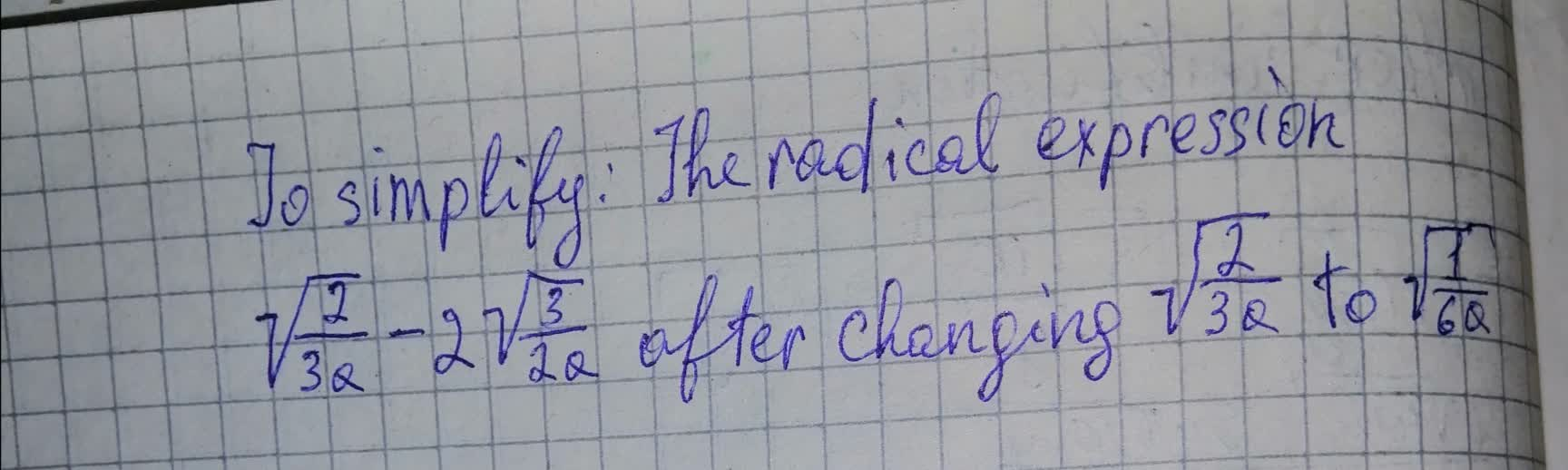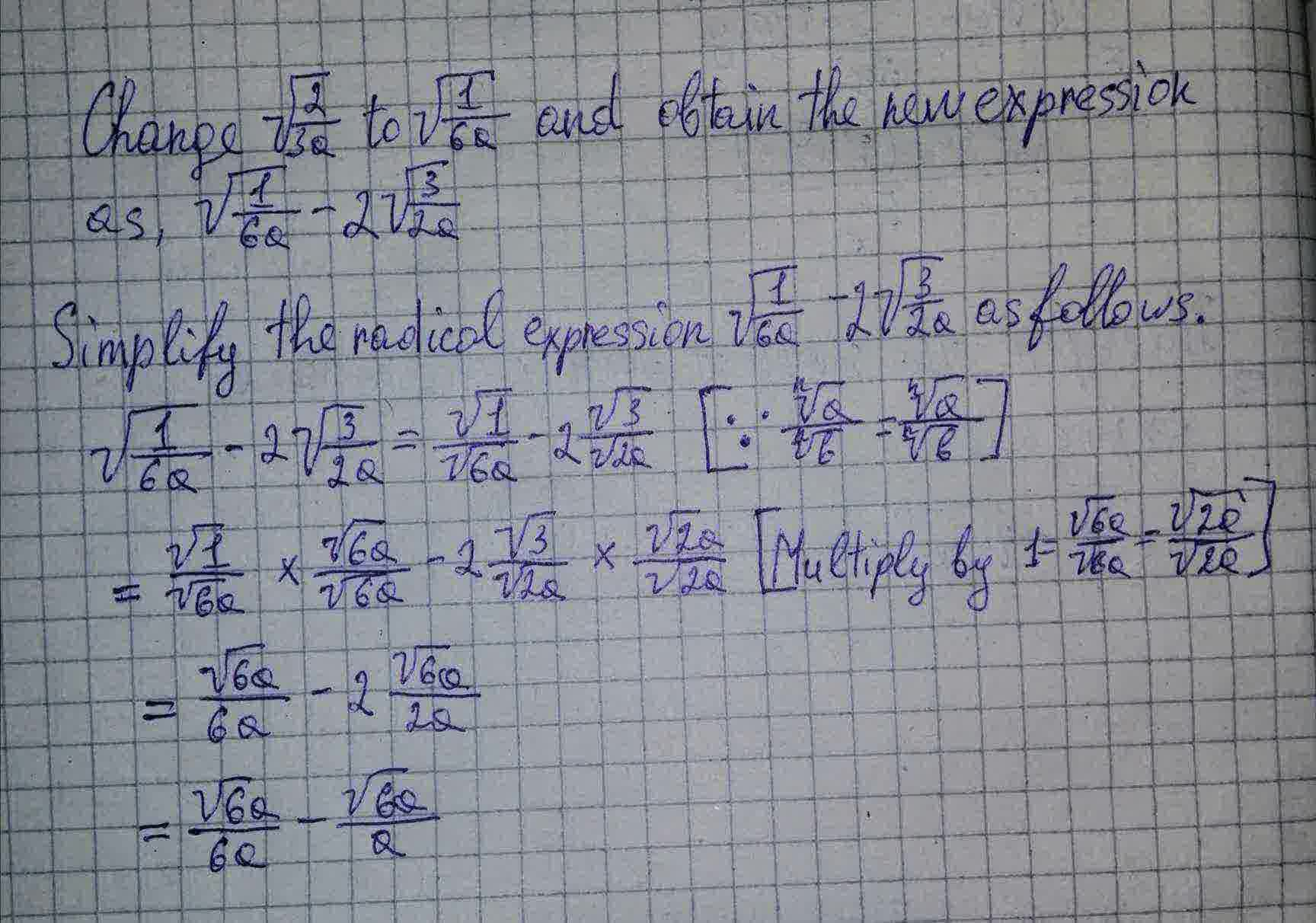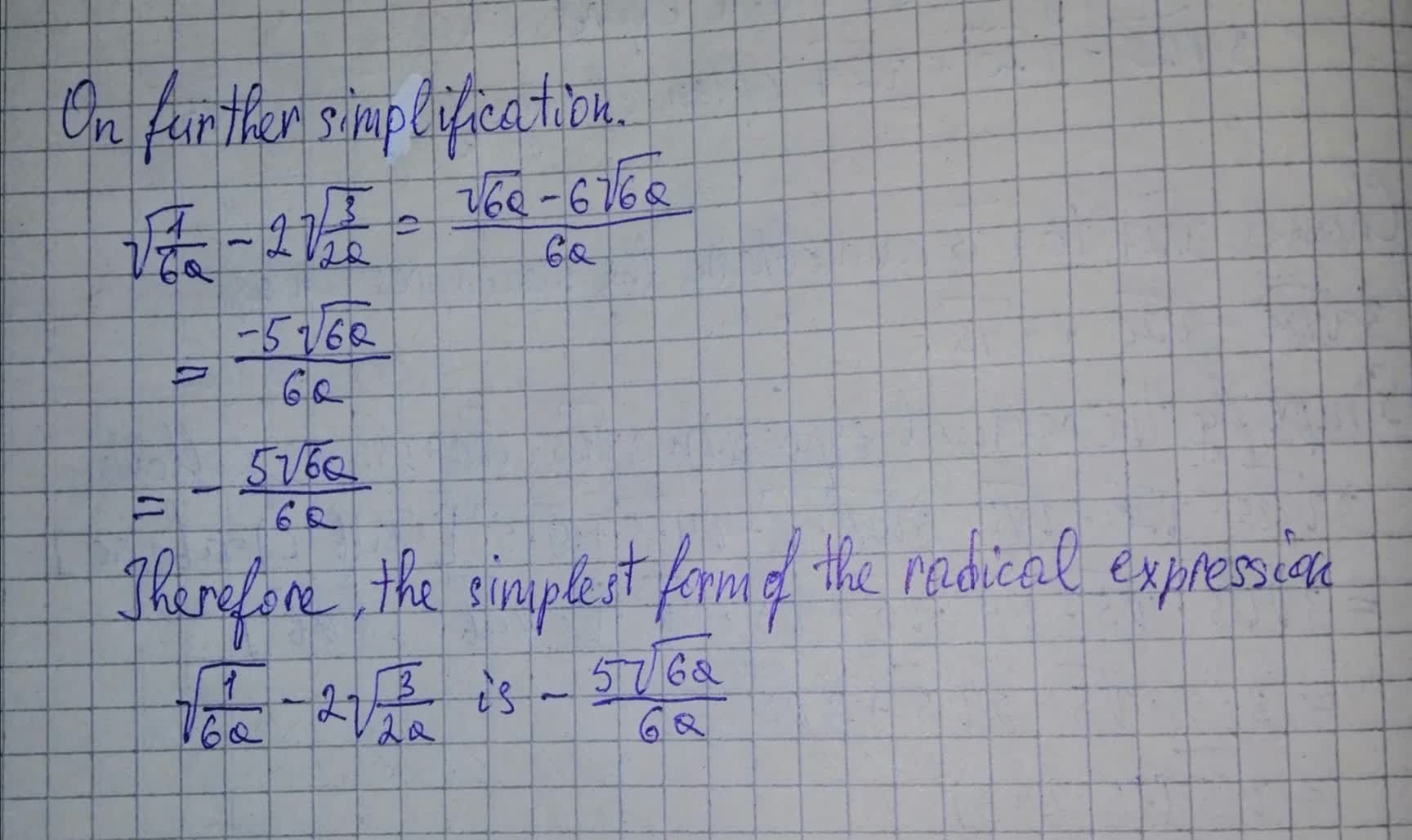emancipezN 2021-07-13 Answered
Simplify radical expression $\sqrt{\frac{2}{3a}}-2\sqrt{\frac{2}{3a}}$You can still ask an expert for help

• Questions are typically answered in as fast as 30 minutes

Solve your problem for the price of one coffee

• Math expert for every subject
• Pay only if we can solve itNola Robson###### Not exactly what you’re looking for?

There is only one solution: $-\sqrt{\frac{2}{3}}\sqrt{\frac{1}{a}}$